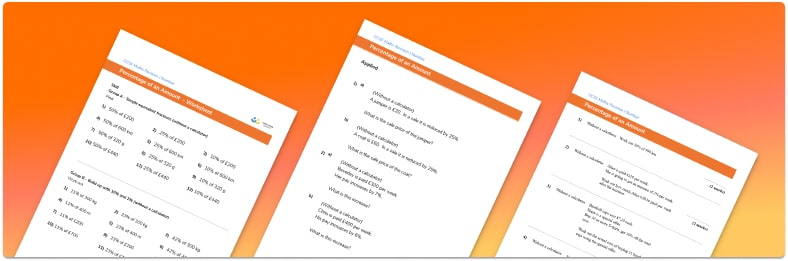# Percentage Of An Amount Worksheet• Section 1 of the percentage of an amount worksheet contains 36 skills-based percentage of an amount questions, in 3 groups to support differentiation
• Section 2 contains 4 applied percentage of an amount questions with a mix of word problems and deeper problem solving questions
• Section 3 contains 4 foundation and higher level GCSE exam style percentage of an amount questions
• Answer sheets and a mark scheme for all percentage of an amount questions are provided
• Questions follow variation theory with plenty of opportunities for students to work independently and solve problems at their own level
• All questions created by fully qualified expert secondary maths teachers

Suitable for GCSE maths revision for AQA, OCR and Edexcel exam boards

• This field is for validation purposes and should be left unchanged.

You can unsubscribe at any time (each email we send will contain an easy way to unsubscribe). To find out more about how we use your data, see our privacy policy.

### Percentage of an amount at a glance

A percentage is an amount expressed as a fraction with a denominator of 100. For example 45% means ‘45 out of 100’, 45 hundredths or 45 over 100. In decimal form, we write 0.45. A percentage does not always have to be a whole number.

There are some simple percentages of amounts that students should be able to calculate mentally, such as 50% (half), 25% (quarter) and 10% (divide by 10). Finding multiples of these amounts, such as 20%, 30% etc. along with bar modelling can be useful to support percentage calculations.

When finding percentages of amounts using a calculator, students should be encouraged to use the decimal equivalent and convert to a decimal multiplication – for example, to find 18% of a given number, multiply by 0.18. Developing this skill is particularly useful when extending to reverse percentage problems, where students can find the original amount by using the inverse operation – so dividing by the percentage decimal equivalent.

Looking forward, students can then progress to additional number worksheets, for example a fractions of amounts worksheet or a. There are plenty of additional percentage problems worksheets, including percentage increase and decrease, and compound interest.For more teaching and learning support on Number our GCSE maths lessons provide step by step support for all GCSE maths concepts.

## Do you have KS4 students who need more focused attention to succeed at GCSE?There will be students in your class who require individual attention to help them succeed in their maths GCSEs. In a class of 30, it’s not always easy to provide.

Help your students feel confident with exam-style questions and the strategies they’ll need to answer them correctly with our dedicated GCSE maths revision programme.

Lessons are selected to provide support where each student needs it most, and specially-trained GCSE maths tutors adapt the pitch and pace of each lesson. This ensures a personalised revision programme that raises grades and boosts confidence.

Find out more